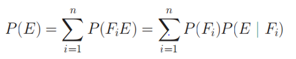# Total Probability Law

## Total Probability Law

Let S be the Sample space and let F1 ,F2………Fn­    be the n mutually exclusive and exhaustive events associated with the random experiments. If E is any event which occurs with those n mutually exclusive events, then## Total Probability Law Solved example

Question
I have three bags that each contain 100 marbles:
Bag A has 75 red and 25 blue marbles;
Bag B has 60 red and 40 blue marbles;
Bag C has 45 red and 55 blue marbles.
I choose one of the bags at random and then pick a marble from the chosen bag, also at random. What is the probability that the chosen marble is red?
Let E be the event that the chosen marble is red. Let Bi be the event that I choose Bag i. We already know that
P (E|BA )=0.75,
P (E|BB )=0.60,
P (E|BC )=0.45 And
P( BA) = P( BA)= P( BA) =1/3
Now the events BA ,BB ,BC   are mutually exclusive events (only one of them can happen), and secondly, because their union is the entire sample space as one the bags will be chosen for sure, i.e., P(BA ∪ BB ∪ BB)=1.
So
Using the law of total probability, we can write
P(E)   = P(E|BA) P( BA) + P(E|BB) P( BB)  + P(E|BC) P( BC) =(0.75)(1/3)+(0.60)(1/3)+(0.45)(1/3)

=0.60

## Related Topics

Go back to Class 12 Main Page using below links